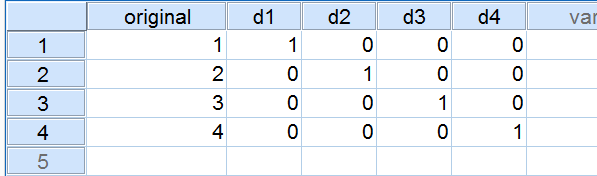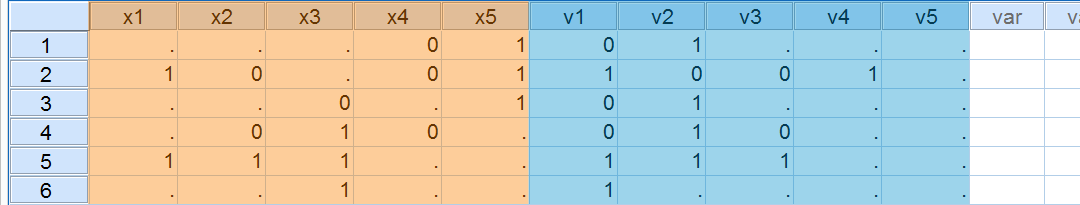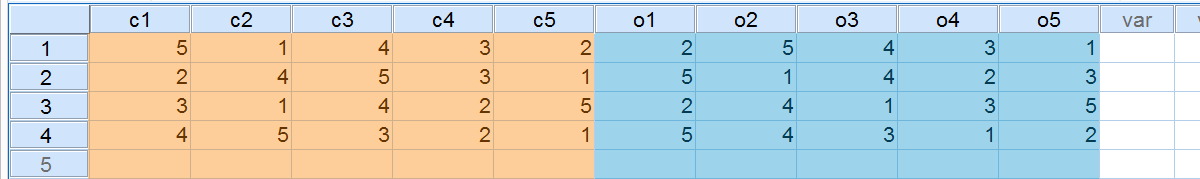SPSS TUTORIALS BASICS ANOVA REGRESSION FACTOR CORRELATION

# SPSS VECTOR – Quick Tutorial

In SPSS, a VECTOR is a list of (new or existing) variables that can be referenced by their indices in this list. VECTOR is often combined with LOOP.Creating Dummy Variables with VECTOR and LOOP

## SPSS Vector - Basic Example

Suppose we'd like to Creating Dummy Variables in SPSS for a variable holding values 1 through 4. We'll call the four dummy variables d1 through d4. Now runningVECTOR d(4).first creates these new (empty) variables. As long as the VECTOR is in effect, d1 can be addressed by d(1) and so on. Let's first run the syntax below to verify this.

## SPSS Vector Syntax Example

*1. Create mini dataset.

data list free/original.
begin data
1 2 3 4
end data.

*2. Define vector for new variables d1 to d4.

vector d(4).

*3. As long as the vector exists, d1 can be addressed as d(1) and so on.

compute d(1) = original = 1.
compute d(2) = original = 2.
compute d(3) = original = 3.
compute d(4) = original = 4.
exe.

## SPSS Vector with Loop

Our first VECTOR syntax didn't save us any effort. So why use VECTOR here? The point is that being able to address variables by their indices enables us to LOOP over them. The syntax below, building upon its predecessor, demonstrates the simplest possible example of this. It deletes the new variables and then recreates them in a more efficient way. Note that we use a scratch variable as our loop index.This COMPUTE command may strike you as odd. It's explained in Compute A = B = C.

## SPSS Vector with Loop Syntax

*1. Delete new variables.

delete variables d1 to d4.

*2. Vector.

vector d(4).

*3. Use vector with loop.

loop #i = 1 to 4.
compute d(#i) = original = #i.
end loop.
exe.

## Fastest Dummification

A little known use of VECTOR is addressing variables by a non constant (over cases). So if we have a variable original,COMPUTE d(original) = 1.generates different COMPUTE commands for different cases. So for a case holding 3 on original, it implies COMPUTE d3 = 1. The other new variables, d1, d2 and d4 are not affected (and thus hold only system missing values but we'll fix that with RECODE).
The syntax below may be the fastest way to create unlabelled dummy variables. However, since it's highly recommended to label new variables and their values, we recommend our Create Dummy Variables tool for practical purposes.

## SPSS Vector Syntax Example

*1. Delete new variables.

delete variables d1 to d4.

*2. Vector.

vector d(4).

*3. Use non constant over cases (variable "original") in vector.

compute d(original) = 1.
exe.

*4. Correct system missings in new variables.

recode d1 to d4 (sysmis = 0).
exe.

## SPSS Vector of Existing Variables

Thus far we used VECTOR for creating new variables. Alternatively, we can use it for addressing existing variables with a slightly different syntax: for addressing the (existing) variables d1 through d4, we'll useVECTOR d = d1 TO d4.We can use this for reversing the aforementioned dummification. The next syntax example demonstrates this by looping over an IF command.

## SPSS Vector Syntax Example

*1. Vector of existing rather than new variables.

vector d = d1 to d4.

*2. Reconstruct multinomial variable from dummy variables.

loop #v = 1 to 4.
if d(#v) = 1 reconstructed = #v.
end loop.
exe.

## SPSS Vector - Final Notes

• Several vectors can be defined at once in a single VECTOR command if desired.
• Those familiar with DO REPEAT will notice that the combination of VECTOR and LOOP offers reasonably similar functionality. A comparison is in place but beyond the scope of this tutorial.
• As a minor technical point, any VECTOR definition stays in effect only until any transformations are run.

# SPSS Vector Bonus Examples

## 1. Shift Values Forward

“I have missing values in my data. I'd like to shift the valid values forward within cases so they become adjacent. How can I accomplish that?Data Before and After Shifting Valid Values Forward

## SPSS Vector Syntax Example

*1. Create dataset.

data list free/x1 to x5.
begin data
'' '' '' 0 1 1 0 '' 0 1 '' '' 0 '' 1 '' 0 1 0 '' 1 1 1 '' '' '' '' 1 '' '' '' '' 0 0 1 1 1 '' '' 1 '' 1 '' 0 '' '' 1 1 '' 0
end data.

*2. Shift values forward.

compute #new = 1.

vector x = x1 to x5 / v(5).
loop #old = 1 to 5.
if not(sysmis(x(#old))) v(#new) = x(#old).
if not(sysmis(x(#old))) #new = #new + 1.
end loop.
exe.

## Explanation

We'll use one vector for the existing variables and one for new variables. We'll loop through the old variables and every time a valid value is encountered, #new increases by 1. Like so, v(#new) may refer to different variables for different cases. However, it always refers to the first new variable that doesn't have a valid value yet. It's this variable that'll take the next valid value we encounter on the old variables.

## 2. Unrank Data

“I asked respondents to rank 5 products. The first variable contains their first choice, the second variable their second and so on. However, I'd like to have a variable per product that has a 1 if it was the first choice, a 2 if it was the second product and so on.”Data Before and After Unranking Values

## SPSS Vector Syntax Example

*1. Create rank data.

data list free/c1 to c5. /*c1 is the first choice, c2 the second and so on.
begin data.
3 4 2 5 1 2 4 5 1 3 1 3 4 5 2 2 5 3 4 1
end data.

*2. Unrank data.

vector o(5)/old = c1 to c5./*o1 is the first option chosen, o2 the second and so on.
loop #value = 1 to 7.
compute o(old(#value)) = #value.
end loop.
exe.

## Explanation

We basically use a vector within a vector within the loop. So the value held by the first variable refers to the vector index of the new variable, which gets value 1. Next, value 2 is passed into the new variable whose index is in c2 and so on.

# Tell us what you think!

*Required field. Your comment will show up after approval from a moderator.

# THIS TUTORIAL HAS 10 COMMENTS:

•### By Ruben Geert van den Berg on December 22nd, 2020

Hi Jerry, great question!

First off, DO REPEAT is probably what you're looking for.

Alternatively, you could perhaps reorder your variables as discussed in SPSS - Reorder Variables with Syntax.

Also note that some commands (such as RECODE) handle multiple variables out of the box.

Let me know if any of these options gets the job done for you, ok?

Kind regards,

Ruben Geert van den Berg
SPSS tutorials

•### By Dick Bierman on May 17th, 2021

Hi Ruben, is there a way to correlate two vectors per case (a part from entering a correlation formula in compute).

I have heavily biased vectors and the actual correlations are the dependent variable in the study. The MCE is not 0 because of their biases. I want to use bootstrap to compare these actual vector correlations with a chance distribution.

Therefore I need to resample the 2 vectors. How would I do that?

Thanks for any hint or example.

Dick (The Netherlands)

•### By Ruben Geert van den Berg on May 17th, 2021

Hi Dick!

Precisely what do you mean by "vector" and "MCE"?

You surely don't seem to refer to an SPSS VECTOR which is simply a shorthand for an array of (new or existing) variables?

Best,

Ruben (also Netherlands)

•### By John on July 4th, 2022

Hi, thank you for the examples!
I am struggling to convert a sparse matrix into a dense one.
Imagine this is the input:
data list free/c1 to c5. /*c1 is rating of first brand, c2 is rating of second brand, etc.
begin data.
, ,1, ,5, ,10, , ,8, , , ,1,7, , ,11, , ,5, ,4,1,
end data.

I need to restructure it to
1,5, , , ,10,8, , , ,1,7, , , ,11, , , , ,5,4,1, ,

Let's assume there are 5 brands and that the rating values are from 1 to 11. I tried using the scipy .todense() module but it gets stuck with no result or error.

•### By Ruben Geert van den Berg on July 5th, 2022

Hi John!

Try this:

vector n(5).
compute #input = 1.
do repeat #oldvars = c1 to c5.
do if(not missing(#oldvars)).
compute n(#input) = #oldvars.
compute #input = #input + 1.
end if.
end repeat.
execute.

Hope that helps!

SPSS tutorials